Published

# SE423 Final Project

Wall riding and obstacle avoiding using IR sensors, bump switches, PI motor control, and T. I Launchpad

IntermediateShowcase (no instructions)10 hours31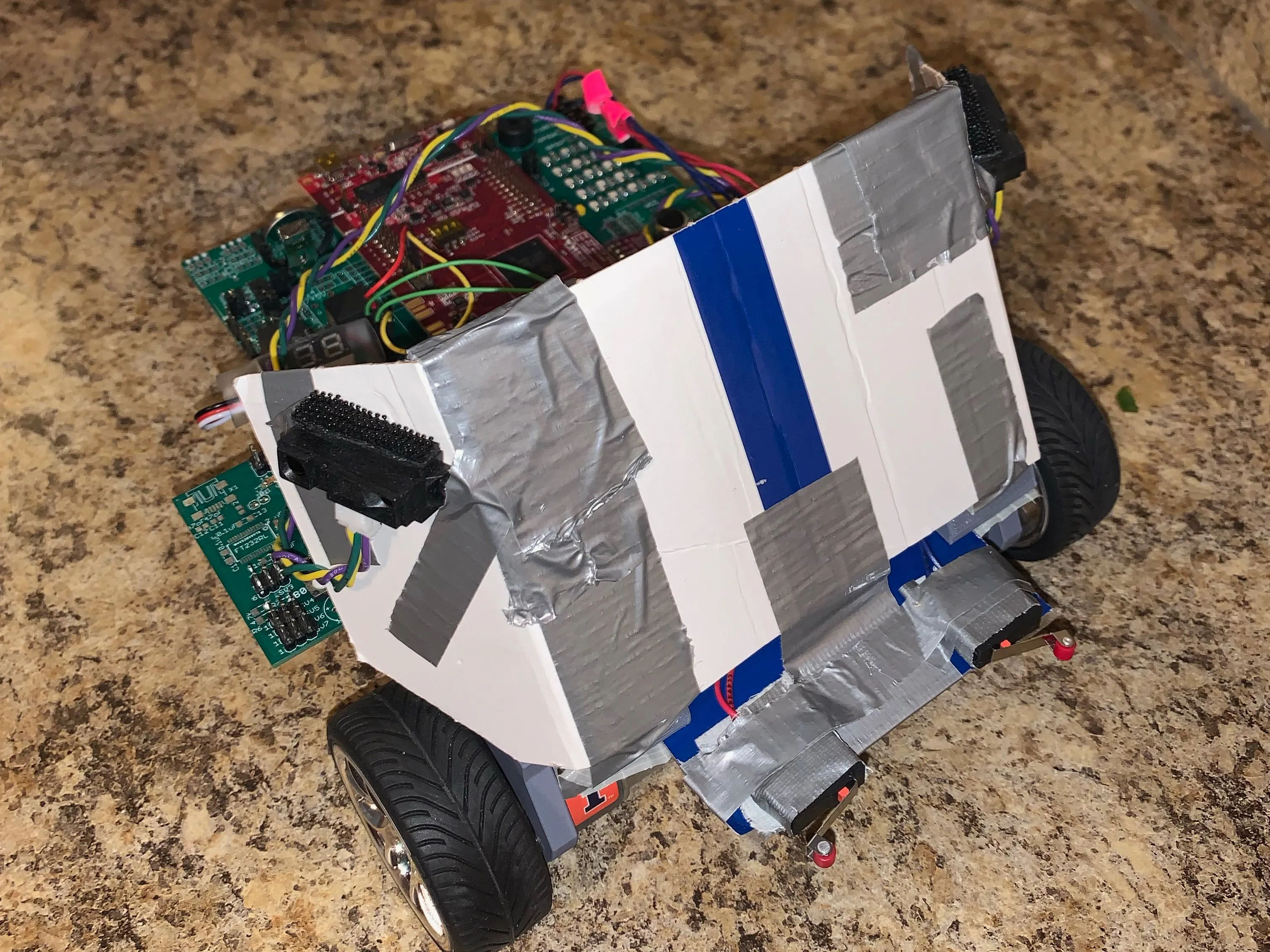## Things used in this project

### Hardware components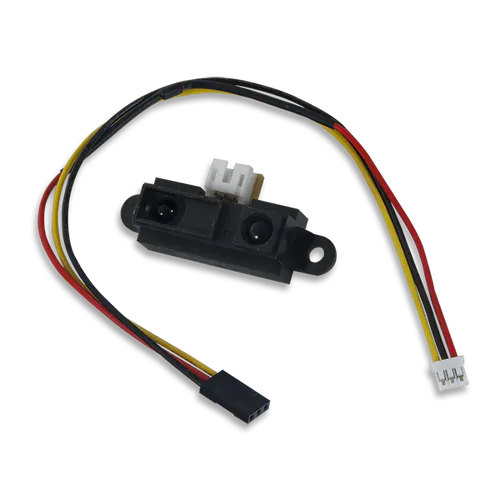Digilent IR Range Sensor
×1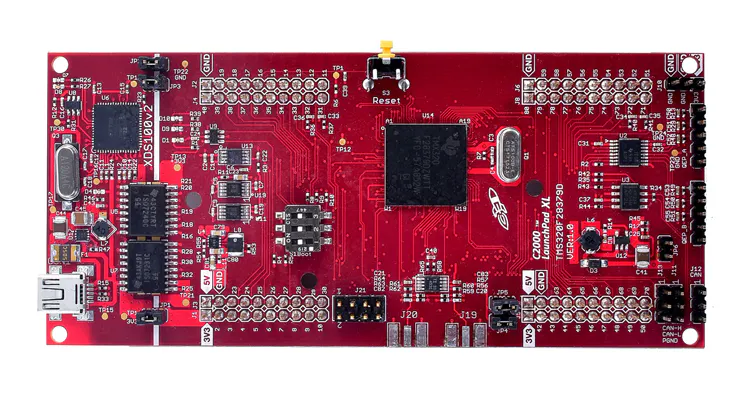Texas Instruments LAUNCHXL-F28379D C2000 Delfino LaunchPad
×1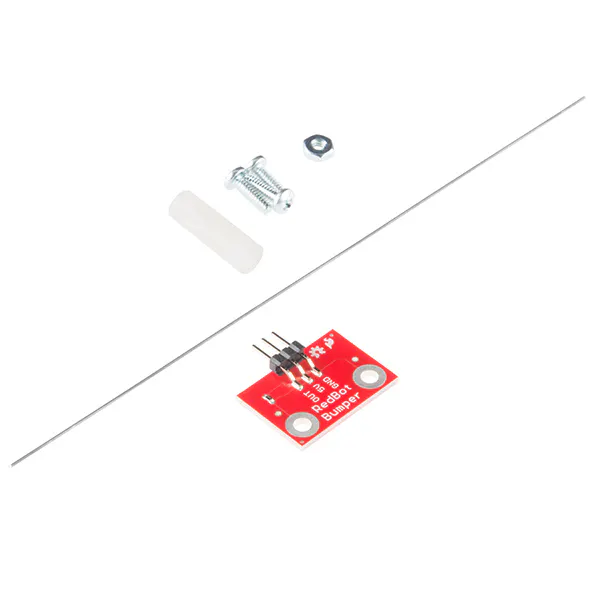SparkFun RedBot Sensor - Mechanical Bumper
×1

### Software apps and online servicesTexas Instruments Code Composer Studio

### Hand tools and fabrication machinesSoldering iron (generic)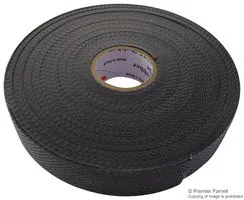Tape, Electrical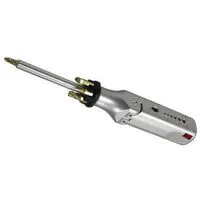Multitool, Screwdriver

Read more

## Schematics

Movie

Movie

### IR Sensor 1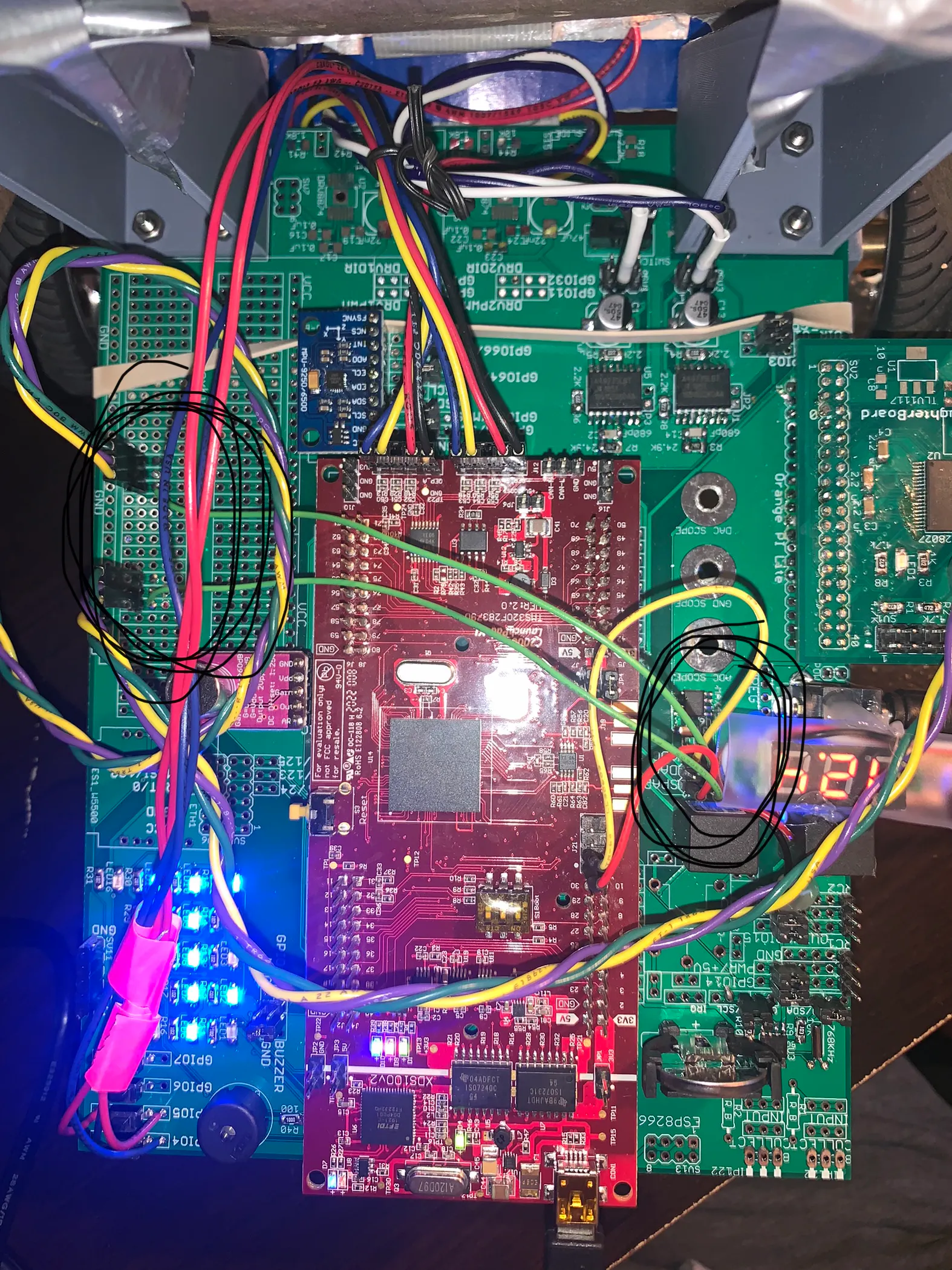### IR Sensor 2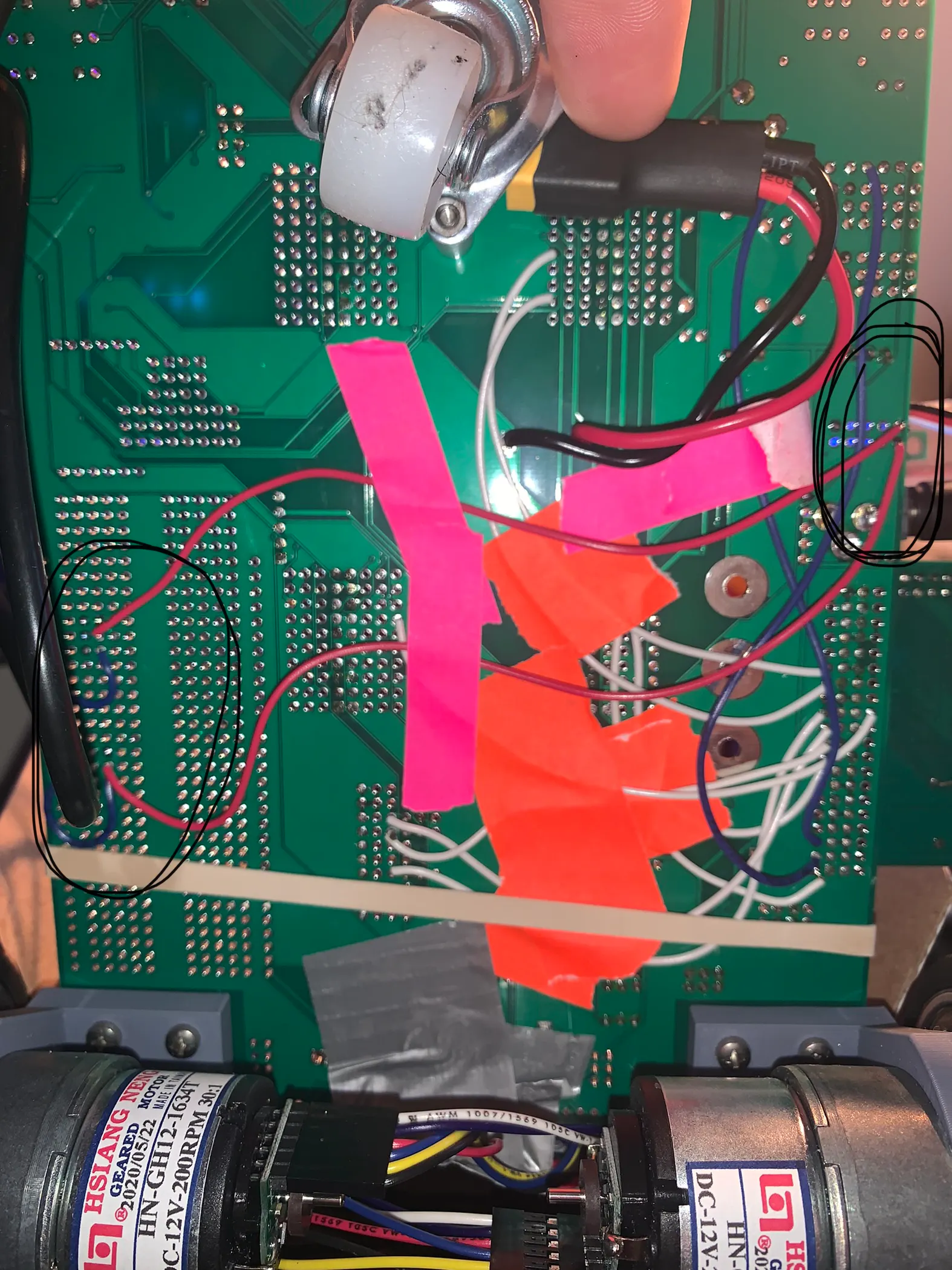### Entire Car Setup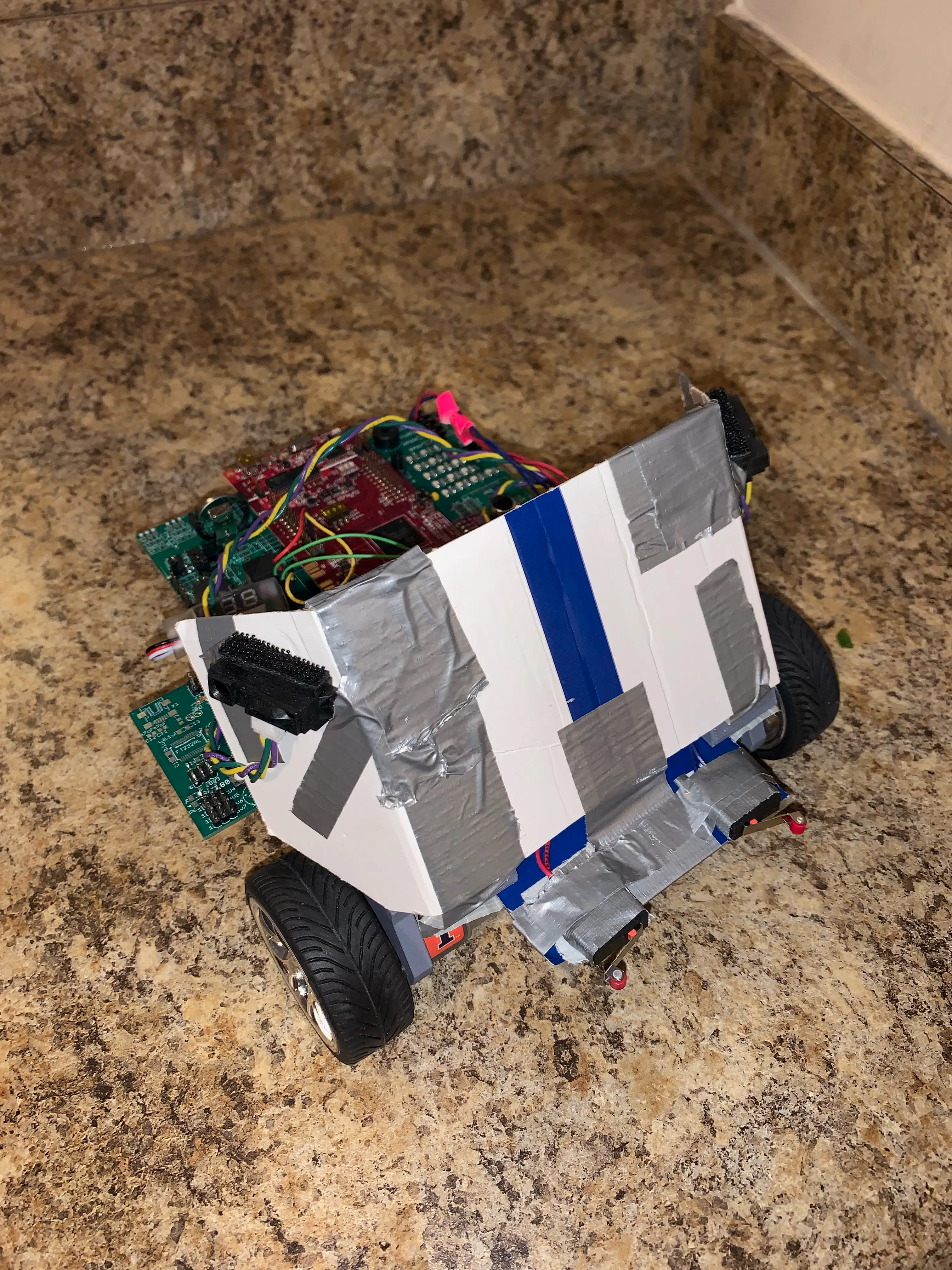## Code

### Main Code

C/C++
```//#############################################################################
// FILE:   Finalv2.c
//
// TITLE:  Butera-Final Project
//Wall-riding and obstacle voiding
//#############################################################################

// Included Files
#include <stdio.h>
#include <stdlib.h>
#include <stdarg.h>
#include <string.h>
#include <math.h>
#include <limits.h>
#include "F28x_Project.h"
#include "driverlib.h"
#include "device.h"
#include "f28379dSerial.h"
#include "LEDPatterns.h"
#include "song.h"
#include "dsp.h"
#include "fpu32/fpu_rfft.h"

#define PI          3.1415926535897932384626433832795
#define TWOPI       6.283185307179586476925286766559
#define HALFPI      1.5707963267948966192313216916398

// Interrupt Service Routines predefinition
__interrupt void cpu_timer0_isr(void);
__interrupt void cpu_timer1_isr(void);
__interrupt void cpu_timer2_isr(void);
__interrupt void SWI_isr(void);
__interrupt void SPIB_isr(void);
void setupSpib(void);
void init_eQEPs(void);
void setEPWM2A(float uLeft);
void setEPWM2B(float uRight);
float readEncLeft(void);
float readEncRight(void);
void serialRXA(serial_t *s, char data);

int16_t ReadSwitches(void);
__interrupt void ADCD_ISR(void);
void setDACA(float);
void setDACB(float);

// Count variables
uint32_t numTimer0calls = 0;
uint32_t numSWIcalls = 0;
uint32_t numRXA = 0;
uint16_t UARTPrint = 0;
uint16_t LEDdisplaynum = 0;
uint32_t increment = 0;
uint32_t timer = 0;

//dummy variables
int16_t temp = 0;
int16_t tempraw = 0;

//Sensor Readings
int16_t updownservoa = 0;
int16_t updownservob = 0;
float ADC1volts = 0;
float ADC2volts = 0;
uint16_t RC1Value = 1500;
uint16_t RC2Value = 1500;
uint16_t updown = 0;
float controlA = 0;
float controlB = 0;
int16_t updownA = 0;
int16_t updownB = 0;
float accelXreading = 0;
float accelYreading = 0;
float accelZreading = 0;
float gyroXreading = 0;
float gyroYreading = 0;
float gyroZreading = 0;

/////Lab6//////
//Motor and position variables
float LeftWheel = 0;
float LeftWheelDist = 0;
float RightWheel = 0;
float RightWheelDist = 0;
float LeftWheelDist_1 = 0;
float RightWheelDist_1 = 0;
float uLeft = 5;
float uRight = 5;
float VLeftK = 0;
float VRightK = 0;
float VRef = .25;
//EX3
float ErrorRightK = 0;
float ErrorRightK_1 = 0;
float ErrorLeftK = 0;
float ErrorLeftK_1 = 0;
float ILeftK = 0;
float ILeftK_1 = 0;
float IRightK = 0;
float IRightK_1 = 0;
float ULeftK = 0;
float URightK = 0;
float Kp = 3;
float Ki = 25;
//EX4
float KT = 3;
float turn = 0;
float et = 0;
//EX5
float Wr = 0.61;
float Rwh = 0.10625;
float thetaR = 0;
float thetaL = 0;
float thetaR_1 = 0;
float thetaL_1 = 0;
float thetaAvg = 0;
float phiR = 0;
float posX = 0;
float posY = 0;
float thetaRD = 0;
float thetaLD = 0;
float thetaAvgD = 0;
float posXD = 0;
float posYD = 0;
float posX_1 = 0;
float posY_1 = 0;
float posX_1D = 0;
float posY_1D = 0;

//Wall Sensor Variables//
int16_t MySwitches = 0;
int16_t adcd0result = 0;
int16_t adcd1result = 0;
float adcd1volts = 0;
float adcd0volts = 0;
float dacouta0 = 0;
float dacouta1 = 0;
uint32_t countvariable;
float rightdist = 0;
float leftdist = 0;
float refdist = 1;
float ewall = 0;
float turns = 0;
float kpwall = 1;

void main(void)
{
// PLL, WatchDog, enable Peripheral Clocks
// This example function is found in the F2837xD_SysCtrl.c file.
InitSysCtrl();

InitGpio();

// Blue LED on LuanchPad
GPIO_SetupPinMux(31, GPIO_MUX_CPU1, 0);
GPIO_SetupPinOptions(31, GPIO_OUTPUT, GPIO_PUSHPULL);
GpioDataRegs.GPASET.bit.GPIO31 = 1;

// Red LED on LaunchPad
GPIO_SetupPinMux(34, GPIO_MUX_CPU1, 0);
GPIO_SetupPinOptions(34, GPIO_OUTPUT, GPIO_PUSHPULL);
GpioDataRegs.GPBSET.bit.GPIO34 = 1;

// LED1 and PWM Pin
GPIO_SetupPinMux(22, GPIO_MUX_CPU1, 0);
GPIO_SetupPinOptions(22, GPIO_OUTPUT, GPIO_PUSHPULL);
GpioDataRegs.GPACLEAR.bit.GPIO22 = 1;

// LED2
GPIO_SetupPinMux(94, GPIO_MUX_CPU1, 0);
GPIO_SetupPinOptions(94, GPIO_OUTPUT, GPIO_PUSHPULL);
GpioDataRegs.GPCCLEAR.bit.GPIO94 = 1;

// LED3
GPIO_SetupPinMux(95, GPIO_MUX_CPU1, 0);
GPIO_SetupPinOptions(95, GPIO_OUTPUT, GPIO_PUSHPULL);
GpioDataRegs.GPCCLEAR.bit.GPIO95 = 1;

// LED4
GPIO_SetupPinMux(97, GPIO_MUX_CPU1, 0);
GPIO_SetupPinOptions(97, GPIO_OUTPUT, GPIO_PUSHPULL);
GpioDataRegs.GPDCLEAR.bit.GPIO97 = 1;

// LED5
GPIO_SetupPinMux(111, GPIO_MUX_CPU1, 0);
GPIO_SetupPinOptions(111, GPIO_OUTPUT, GPIO_PUSHPULL);
GpioDataRegs.GPDCLEAR.bit.GPIO111 = 1;

// LED6
GPIO_SetupPinMux(130, GPIO_MUX_CPU1, 0);
GPIO_SetupPinOptions(130, GPIO_OUTPUT, GPIO_PUSHPULL);
GpioDataRegs.GPECLEAR.bit.GPIO130 = 1;

// LED7
GPIO_SetupPinMux(131, GPIO_MUX_CPU1, 0);
GPIO_SetupPinOptions(131, GPIO_OUTPUT, GPIO_PUSHPULL);
GpioDataRegs.GPECLEAR.bit.GPIO131 = 1;

// LED8
GPIO_SetupPinMux(25, GPIO_MUX_CPU1, 0);
GPIO_SetupPinOptions(25, GPIO_OUTPUT, GPIO_PUSHPULL);
GpioDataRegs.GPACLEAR.bit.GPIO25 = 1;

// LED9
GPIO_SetupPinMux(26, GPIO_MUX_CPU1, 0);
GPIO_SetupPinOptions(26, GPIO_OUTPUT, GPIO_PUSHPULL);
GpioDataRegs.GPACLEAR.bit.GPIO26 = 1;

// LED10
GPIO_SetupPinMux(27, GPIO_MUX_CPU1, 0);
GPIO_SetupPinOptions(27, GPIO_OUTPUT, GPIO_PUSHPULL);
GpioDataRegs.GPACLEAR.bit.GPIO27 = 1;

// LED11
GPIO_SetupPinMux(60, GPIO_MUX_CPU1, 0);
GPIO_SetupPinOptions(60, GPIO_OUTPUT, GPIO_PUSHPULL);
GpioDataRegs.GPBCLEAR.bit.GPIO60 = 1;

// LED12
GPIO_SetupPinMux(61, GPIO_MUX_CPU1, 0);
GPIO_SetupPinOptions(61, GPIO_OUTPUT, GPIO_PUSHPULL);
GpioDataRegs.GPBCLEAR.bit.GPIO61 = 1;

// LED13
GPIO_SetupPinMux(157, GPIO_MUX_CPU1, 0);
GPIO_SetupPinOptions(157, GPIO_OUTPUT, GPIO_PUSHPULL);
GpioDataRegs.GPECLEAR.bit.GPIO157 = 1;

// LED14
GPIO_SetupPinMux(158, GPIO_MUX_CPU1, 0);
GPIO_SetupPinOptions(158, GPIO_OUTPUT, GPIO_PUSHPULL);
GpioDataRegs.GPECLEAR.bit.GPIO158 = 1;

// LED15
GPIO_SetupPinMux(159, GPIO_MUX_CPU1, 0);
GPIO_SetupPinOptions(159, GPIO_OUTPUT, GPIO_PUSHPULL);
GpioDataRegs.GPECLEAR.bit.GPIO159 = 1;

// LED16
GPIO_SetupPinMux(160, GPIO_MUX_CPU1, 0);
GPIO_SetupPinOptions(160, GPIO_OUTPUT, GPIO_PUSHPULL);
GpioDataRegs.GPFCLEAR.bit.GPIO160 = 1;

//WIZNET Reset
GPIO_SetupPinMux(0, GPIO_MUX_CPU1, 0);
GPIO_SetupPinOptions(0, GPIO_OUTPUT, GPIO_PUSHPULL);
GpioDataRegs.GPASET.bit.GPIO0 = 1;

//ESP8266 Reset
GPIO_SetupPinMux(1, GPIO_MUX_CPU1, 0);
GPIO_SetupPinOptions(1, GPIO_OUTPUT, GPIO_PUSHPULL);
GpioDataRegs.GPASET.bit.GPIO1 = 1;

//SPIRAM  CS  Chip Select
GPIO_SetupPinMux(19, GPIO_MUX_CPU1, 0);
GPIO_SetupPinOptions(19, GPIO_OUTPUT, GPIO_PUSHPULL);
GpioDataRegs.GPASET.bit.GPIO19 = 1;

//DRV8874 #1 DIR  Direction
GPIO_SetupPinMux(29, GPIO_MUX_CPU1, 0);
GPIO_SetupPinOptions(29, GPIO_OUTPUT, GPIO_PUSHPULL);
GpioDataRegs.GPASET.bit.GPIO29 = 1;

//DRV8874 #2 DIR  Direction
GPIO_SetupPinMux(32, GPIO_MUX_CPU1, 0);
GPIO_SetupPinOptions(32, GPIO_OUTPUT, GPIO_PUSHPULL);
GpioDataRegs.GPBSET.bit.GPIO32 = 1;

//DAN28027  CS  Chip Select
GPIO_SetupPinMux(9, GPIO_MUX_CPU1, 0);
GPIO_SetupPinOptions(9, GPIO_OUTPUT, GPIO_PUSHPULL);
GpioDataRegs.GPASET.bit.GPIO9 = 1;

//MPU9250  CS  Chip Select
GPIO_SetupPinMux(66, GPIO_MUX_CPU1, 0);
GPIO_SetupPinOptions(66, GPIO_OUTPUT, GPIO_PUSHPULL);
GpioDataRegs.GPCSET.bit.GPIO66 = 1;

//WIZNET  CS  Chip Select
GPIO_SetupPinMux(125, GPIO_MUX_CPU1, 0);
GPIO_SetupPinOptions(125, GPIO_OUTPUT, GPIO_PUSHPULL);
GpioDataRegs.GPDSET.bit.GPIO125 = 1;

//PushButton 1
GPIO_SetupPinMux(4, GPIO_MUX_CPU1, 0);
GPIO_SetupPinOptions(4, GPIO_INPUT, GPIO_PULLUP);

//PushButton 2
GPIO_SetupPinMux(5, GPIO_MUX_CPU1, 0);
GPIO_SetupPinOptions(5, GPIO_INPUT, GPIO_PULLUP);

//PushButton 3
GPIO_SetupPinMux(6, GPIO_MUX_CPU1, 0);
GPIO_SetupPinOptions(6, GPIO_INPUT, GPIO_PULLUP);

//PushButton 4
GPIO_SetupPinMux(7, GPIO_MUX_CPU1, 0);
GPIO_SetupPinOptions(7, GPIO_INPUT, GPIO_PULLUP);

//Joy Stick Pushbutton
GPIO_SetupPinMux(8, GPIO_MUX_CPU1, 0);
GPIO_SetupPinOptions(8, GPIO_INPUT, GPIO_PULLUP);

// Clear all interrupts and initialize PIE vector table:
// Disable CPU interrupts
DINT;

// Initialize the PIE control registers to their default state.
// The default state is all PIE interrupts disabled and flags
// are cleared.
// This function is found in the F2837xD_PieCtrl.c file.
InitPieCtrl();

// Disable CPU interrupts and clear all CPU interrupt flags:
IER = 0x0000;
IFR = 0x0000;

// Initialize the PIE vector table with pointers to the shell Interrupt
// Service Routines (ISR).
// This will populate the entire table, even if the interrupt
// is not used in this example.  This is useful for debug purposes.
// The shell ISR routines are found in F2837xD_DefaultIsr.c.
// This function is found in F2837xD_PieVect.c.
InitPieVectTable();

// Interrupts that are used in this example are re-mapped to
// ISR functions found within this project
EALLOW;  // This is needed to write to EALLOW protected registers
PieVectTable.TIMER0_INT = &cpu_timer0_isr;
PieVectTable.TIMER1_INT = &cpu_timer1_isr;
PieVectTable.TIMER2_INT = &cpu_timer2_isr;
PieVectTable.SCIA_RX_INT = &RXAINT_recv_ready;
PieVectTable.SCIC_RX_INT = &RXCINT_recv_ready;
PieVectTable.SCID_RX_INT = &RXDINT_recv_ready;
PieVectTable.SCIA_TX_INT = &TXAINT_data_sent;
PieVectTable.SCIC_TX_INT = &TXCINT_data_sent;
PieVectTable.SCID_TX_INT = &TXDINT_data_sent;
PieVectTable.ADCD1_INT = &ADCD_ISR;

PieVectTable.EMIF_ERROR_INT = &SWI_isr;
PieVectTable.SPIB_RX_INT = &SPIB_isr;
EDIS;

EALLOW; // Below are protected registers
GpioCtrlRegs.GPAPUD.bit.GPIO2 = 1; // For EPWM2A
GpioCtrlRegs.GPAPUD.bit.GPIO3 = 1; // For EPWM2B
EDIS;    // This is needed to disable write to EALLOW protected registers

//EPWM2A
//motor function regs
EPwm2Regs.TBCTL.bit.CTRMODE = 0;
EPwm2Regs.TBCTL.bit.CLKDIV = 0;
EPwm2Regs.TBCTL.bit.FREE_SOFT = 0x2;
EPwm2Regs.TBCTL.bit.PHSEN =0;
EPwm2Regs.TBCTR = 0x0;
EPwm2Regs.TBPRD = 2500;
EPwm2Regs.CMPA.bit.CMPA = 2500;
EPwm2Regs.AQCTLA.bit.CAU= 0x1;
EPwm2Regs.AQCTLA.bit.ZRO= 0x2;
EPwm2Regs.TBPHS.bit.TBPHS =0;
GPIO_SetupPinMux(2, GPIO_MUX_CPU1, 1);
//EPWM2B
EPwm2Regs.CMPB.bit.CMPB = 0;
EPwm2Regs.AQCTLB.bit.CBU= 0x1;
EPwm2Regs.AQCTLB.bit.ZRO= 0x2;
GPIO_SetupPinMux(3, GPIO_MUX_CPU1, 1);

EPwm5Regs.ETSEL.bit.SOCAEN = 0; // Disable SOC on A group
EPwm5Regs.TBCTL.bit.CTRMODE = 3; // freeze counter
EPwm5Regs.ETSEL.bit.SOCASEL = 0x2; // Select Event when counter equal to PRD
EPwm5Regs.ETPS.bit.SOCAPRD = 01; // Generate pulse on 1st event (“pulse” is the same as “trigger”)
EPwm5Regs.TBCTR = 0x0; // Clear counter
EPwm5Regs.TBPHS.bit.TBPHS = 0x0000; // Phase is 0
EPwm5Regs.TBCTL.bit.PHSEN = 0; // Disable phase loading
EPwm5Regs.TBCTL.bit.CLKDIV = 0; // divide by 1 50Mhz Clock
//    EPwm5Regs.TBPRD = 50000; // Set Period to 1ms sample. Input clock is 50MHz. ??????
EPwm5Regs.TBPRD = 5000; // Set Period to .1ms sample. Input clock is 50MHz. ??????
// Notice here that we are not setting CMPA or CMPB because we are not using the PWM signal
EPwm5Regs.ETSEL.bit.SOCAEN = 1; //enable SOCA
EPwm5Regs.TBCTL.bit.CTRMODE = 0; //unfreeze, and enter up

EALLOW;
//write configurations for all ADCs ADCA, ADCB, ADCC, ADCD
AdcaRegs.ADCCTL2.bit.PRESCALE = 6; //set ADCCLK divider to /4
AdcbRegs.ADCCTL2.bit.PRESCALE = 6; //set ADCCLK divider to /4
AdccRegs.ADCCTL2.bit.PRESCALE = 6; //set ADCCLK divider to /4
AdcdRegs.ADCCTL2.bit.PRESCALE = 6; //set ADCCLK divider to /4
AdcSetMode(ADC_ADCA, ADC_RESOLUTION_12BIT, ADC_SIGNALMODE_SINGLE); //read calibration settings
AdcSetMode(ADC_ADCB, ADC_RESOLUTION_12BIT, ADC_SIGNALMODE_SINGLE); //read calibration settings
AdcSetMode(ADC_ADCC, ADC_RESOLUTION_12BIT, ADC_SIGNALMODE_SINGLE); //read calibration settings
AdcSetMode(ADC_ADCD, ADC_RESOLUTION_12BIT, ADC_SIGNALMODE_SINGLE); //read calibration settings
//Set pulse positions to late
AdcaRegs.ADCCTL1.bit.INTPULSEPOS = 1;
AdcbRegs.ADCCTL1.bit.INTPULSEPOS = 1;
AdccRegs.ADCCTL1.bit.INTPULSEPOS = 1;
AdcdRegs.ADCCTL1.bit.INTPULSEPOS = 1;
//power up the ADCs
AdcaRegs.ADCCTL1.bit.ADCPWDNZ = 1;
AdcbRegs.ADCCTL1.bit.ADCPWDNZ = 1;
AdccRegs.ADCCTL1.bit.ADCPWDNZ = 1;
AdcdRegs.ADCCTL1.bit.ADCPWDNZ = 1;
//delay for 1ms to allow ADC time to power up
DELAY_US(1000);
//Select the channels to convert and end of conversion flag
//Many statements commented out, To be used when using ADCA or ADCB
//ADCA//////
AdcaRegs.ADCSOC0CTL.bit.CHSEL = 0x2; //SOC0 will convert Channel you choose Does not have to be A0
AdcaRegs.ADCSOC0CTL.bit.ACQPS = 99; //sample window is acqps + 1 SYSCLK cycles = 500ns
AdcaRegs.ADCSOC0CTL.bit.TRIGSEL = 0xD;// EPWM5 ADCSOCA or another trigger you choose will trigger SOC0
AdcaRegs.ADCSOC1CTL.bit.CHSEL = 0x3; //SOC1 will convert Channel you choose Does not have to be A1
AdcaRegs.ADCSOC1CTL.bit.ACQPS = 99; //sample window is acqps + 1 SYSCLK cycles = 500ns
AdcaRegs.ADCSOC1CTL.bit.TRIGSEL = 0xD;// EPWM5 ADCSOCA or another trigger you choose will trigger SOC1
AdcaRegs.ADCINTSEL1N2.bit.INT1SEL = 1; //set to last SOC that is converted and it will set INT1 flag ADCA1
AdcaRegs.ADCINTSEL1N2.bit.INT1E = 1; //enable INT1 flag
AdcaRegs.ADCINTFLGCLR.bit.ADCINT1 = 1; //make sure INT1 flag is cleared
//ADCB
AdcbRegs.ADCSOC0CTL.bit.CHSEL = 4; //SOC0 will convert Channel you choose Does not have to be B0
AdcbRegs.ADCSOC0CTL.bit.ACQPS = 99; //sample window is acqps + 1 SYSCLK cycles = 500ns
AdcbRegs.ADCSOC0CTL.bit.TRIGSEL = 0xD;// EPWM5 ADCSOCA or another trigger you choose will trigger SOC0
//AdcbRegs.ADCSOC1CTL.bit.CHSEL = ???; //SOC1 will convert Channel you choose Does not have to be B1
//AdcbRegs.ADCSOC1CTL.bit.ACQPS = 99; //sample window is acqps + 1 SYSCLK cycles = 500ns
//AdcbRegs.ADCSOC1CTL.bit.TRIGSEL = ???;// EPWM5 ADCSOCA or another trigger you choose will trigger SOC1
//AdcbRegs.ADCSOC2CTL.bit.CHSEL = ???; //SOC2 will convert Channel you choose Does not have to be B2
//AdcbRegs.ADCSOC2CTL.bit.ACQPS = 99; //sample window is acqps + 1 SYSCLK cycles = 500ns
//AdcbRegs.ADCSOC2CTL.bit.TRIGSEL = ???;// EPWM5 ADCSOCA or another trigger you choose will trigger SOC2
//AdcbRegs.ADCSOC3CTL.bit.CHSEL = ???; //SOC3 will convert Channel you choose Does not have to be B3
//AdcbRegs.ADCSOC3CTL.bit.ACQPS = 99; //sample window is acqps + 1 SYSCLK cycles = 500ns
//AdcbRegs.ADCSOC3CTL.bit.TRIGSEL = ???;// EPWM5 ADCSOCA or another trigger you choose will trigger SOC3
AdcbRegs.ADCINTSEL1N2.bit.INT1SEL = 0; //set to last SOC that is converted and it will set INT1 flag ADCB1
AdcbRegs.ADCINTSEL1N2.bit.INT1E = 1; //enable INT1 flag
AdcbRegs.ADCINTFLGCLR.bit.ADCINT1 = 1; //make sure INT1 flag is cleared
//ADCD
AdcdRegs.ADCSOC0CTL.bit.CHSEL = 0; // set SOC0 to convert pin D0
AdcdRegs.ADCSOC0CTL.bit.ACQPS = 99; //sample window is acqps + 1 SYSCLK cycles = 500ns
AdcdRegs.ADCSOC0CTL.bit.TRIGSEL = 0xD; // EPWM5 ADCSOCA will trigger SOC0
AdcdRegs.ADCSOC1CTL.bit.CHSEL = 0x1; //set SOC1 to convert pin D1
AdcdRegs.ADCSOC1CTL.bit.ACQPS = 99; //sample window is acqps + 1 SYSCLK cycles = 500ns
AdcdRegs.ADCSOC1CTL.bit.TRIGSEL = 0xD; // EPWM5 ADCSOCA will trigger SOC1
//AdcdRegs.ADCSOC2CTL.bit.CHSEL = ???; //set SOC2 to convert pin D2
//AdcdRegs.ADCSOC2CTL.bit.ACQPS = 99; //sample window is acqps + 1 SYSCLK cycles = 500ns
//AdcdRegs.ADCSOC2CTL.bit.TRIGSEL = ???; // EPWM5 ADCSOCA will trigger SOC2
//AdcdRegs.ADCSOC3CTL.bit.CHSEL = ???; //set SOC3 to convert pin D3
//AdcdRegs.ADCSOC3CTL.bit.ACQPS = 99; //sample window is acqps + 1 SYSCLK cycles = 500ns
//AdcdRegs.ADCSOC3CTL.bit.TRIGSEL = ???; // EPWM5 ADCSOCA will trigger SOC3
AdcdRegs.ADCINTSEL1N2.bit.INT1SEL = 1; //set to SOC1, the last converted, and it will set INT1 flag ADCD1
AdcdRegs.ADCINTSEL1N2.bit.INT1E = 1; //enable INT1 flag
AdcdRegs.ADCINTFLGCLR.bit.ADCINT1 = 1; //make sure INT1 flag is cleared
EDIS;

// Enable DACA and DACB outputs
EALLOW;
DacaRegs.DACOUTEN.bit.DACOUTEN = 1;//enable dacA output-->uses ADCINA0
DacaRegs.DACCTL.bit.LOADMODE = 0;//load on next sysclk
DacaRegs.DACCTL.bit.DACREFSEL = 1;//use ADC VREF as reference voltage
DacbRegs.DACOUTEN.bit.DACOUTEN = 1;//enable dacB output-->uses ADCINA1
DacbRegs.DACCTL.bit.LOADMODE = 0;//load on next sysclk
DacbRegs.DACCTL.bit.DACREFSEL = 1;//use ADC VREF as reference voltage
EDIS;

// Initialize the CpuTimers Device Peripheral. This function can be
// found in F2837xD_CpuTimers.c
InitCpuTimers();

// Configure CPU-Timer 0, 1, and 2 to interrupt every second:
// 200MHz CPU Freq, 1 second Period (in uSeconds)
ConfigCpuTimer(&CpuTimer0, 200, 1000);
ConfigCpuTimer(&CpuTimer1, 200, 20000);
ConfigCpuTimer(&CpuTimer2, 200, 4000);

// Enable CpuTimer Interrupt bit TIE
CpuTimer0Regs.TCR.all = 0x4000;
CpuTimer1Regs.TCR.all = 0x4000;
CpuTimer2Regs.TCR.all = 0x4000;

init_serial(&SerialA,115200,serialRXA);
//    init_serial(&SerialC,115200,serialRXC);
//    init_serial(&SerialD,115200,serialRXD);

setupSpib();
init_eQEPs();
//    setEPWM2A(uLeft);
//    setEPWM2B(uRight);
// ---------------------------------------------------------------------------

IER |= M_INT1;
IER |= M_INT8;  // SCIC SCID
IER |= M_INT9;  // SCIA
IER |= M_INT12;
IER |= M_INT13;
IER |= M_INT14;
IER |= M_INT6;

// Enable TINT0 in the PIE: Group 1 interrupt 7
PieCtrlRegs.PIEIER1.bit.INTx7 = 1;
// Enable SWI in the PIE: Group 12 interrupt 9
PieCtrlRegs.PIEIER12.bit.INTx9 = 1;
//Exercise2 SPIB
PieCtrlRegs.PIEIER6.bit.INTx3 = 1;
PieCtrlRegs.PIEIER1.bit.INTx6 = 1;

// Enable global Interrupts and higher priority real-time debug events
EINT;  // Enable Global interrupt INTM
ERTM;  // Enable Global realtime interrupt DBGM

// IDLE loop. Just sit and loop forever (optional):

while(1)
{
if (UARTPrint == 1 ) {
//serial_printf(&SerialA,"RightWheelVel: %.3f LeftWheelVel: %.3f\r\n",VRightK, VLeftK);
//serial_printf(&SerialA,"AccelX: %.4f AccelY: %.4f AccelZ: %.4f GyroX: %.4f GyroY: %.4f GyroZ: %.4f\r\n",accelXreading,accelYreading,accelZreading,gyroXreading,gyroYreading,gyroZreading);
//serial_printf(&SerialA," PosX: %.2f PosY: %.2f Bearing: %.2f\r\n",posX,posY,phiR);
serial_printf(&SerialA,"Volt RightDist :%f Volt LeftDist:%f\r\n", rightdist, leftdist);
UARTPrint = 0;
}
}
}

int16_t ReadSwitches(void){
int16_t switchvariable = 0;
if (GpioDataRegs.GPADAT.bit.GPIO4 == 0) {
switchvariable = switchvariable|0x1;
//GPIO4 is High/3.3V
}
if (GpioDataRegs.GPADAT.bit.GPIO5 == 0) {
switchvariable = switchvariable|0x2;
//GPIO5 is High/3.3V
}
return switchvariable;
}

//Exercise4
void setupSpib(void) //Call this function in main() somewhere after the DINT; line of code.
{

GPIO_SetupPinMux(9, GPIO_MUX_CPU1, 0); // Set as GPIO9 and used as DAN28027 SS
GPIO_SetupPinOptions(9, GPIO_OUTPUT, GPIO_PUSHPULL); // Make GPIO9 an Output Pin
GpioDataRegs.GPASET.bit.GPIO9 = 1; //Initially Set GPIO9/SS High so DAN28027 is not selected
GPIO_SetupPinMux(66, GPIO_MUX_CPU1, 0); // Set as GPIO66 and used as MPU-9250 SS
GPIO_SetupPinOptions(66, GPIO_OUTPUT, GPIO_PUSHPULL); // Make GPIO66 an Output Pin
GpioDataRegs.GPCSET.bit.GPIO66 = 1; //Initially Set GPIO66/SS High so MPU-9250 is not selected
GPIO_SetupPinMux(63, GPIO_MUX_CPU1, 15); //Set GPIO63 pin to SPISIMOB
GPIO_SetupPinMux(64, GPIO_MUX_CPU1, 15); //Set GPIO64 pin to SPISOMIB
GPIO_SetupPinMux(65, GPIO_MUX_CPU1, 15); //Set GPIO65 pin to SPICLKB

EALLOW;
GpioCtrlRegs.GPBPUD.bit.GPIO63 = 0; // Enable Pull-ups on SPI PINs Recommended by TI for SPI Pins
GpioCtrlRegs.GPCPUD.bit.GPIO64 = 0;
GpioCtrlRegs.GPCPUD.bit.GPIO65 = 0;
GpioCtrlRegs.GPBQSEL2.bit.GPIO63 = 3; // Set I/O pin to asynchronous mode recommended for SPI
GpioCtrlRegs.GPCQSEL1.bit.GPIO64 = 3; // Set I/O pin to asynchronous mode recommended for SPI
GpioCtrlRegs.GPCQSEL1.bit.GPIO65 = 3; // Set I/O pin to asynchronous mode recommended for SPI
EDIS;
//Step 1.
// cut and paste here all the SpibRegs initializations you found for part 3. Make sure the TXdelay in
//between each transfer to 0. Also don’t forget to cut and paste the GPIO settings for GPIO9, 63, 64, 65,
//66 which are also a part of the SPIB setup.
SpibRegs.SPICCR.bit.SPICHAR = 0xF;
SpibRegs.SPIFFCT.bit.TXDLY = 0x00;
SpibRegs.SPICCR.bit.SPISWRESET = 1; // Put SPI in Reset
SpibRegs.SPICTL.bit.CLK_PHASE = 1; //This happens to be the mode for both the DAN28027 and
SpibRegs.SPICCR.bit.CLKPOLARITY = 0; //The MPU-9250, Mode 01.
SpibRegs.SPICTL.bit.MASTER_SLAVE = 1; // Set to SPI Master
SpibRegs.SPICCR.bit.SPICHAR = 0xF; // Set to transmit and receive 16 bits each write to SPITXBUF
SpibRegs.SPICTL.bit.TALK = 1; // Enable transmission
SpibRegs.SPIPRI.bit.FREE = 1; // Free run, continue SPI operation
SpibRegs.SPICTL.bit.SPIINTENA = 0; // Disables the SPI interrupt
SpibRegs.SPIBRR.bit.SPI_BIT_RATE = 0x32; // Set SCLK bit rate to 1 MHz so 1us period. SPI base clock is
// 50MHZ. And this setting divides that base clock to create SCLK’s period
SpibRegs.SPISTS.all = 0x0000; // Clear status flags just in case they are set for some reason
SpibRegs.SPIFFTX.bit.SPIRST = 1;// Pull SPI FIFO out of reset, SPI FIFO can resume transmit or receive.
SpibRegs.SPIFFTX.bit.SPIFFENA = 1; // Enable SPI FIFO enhancements
SpibRegs.SPIFFTX.bit.TXFIFO = 0; // Write 0 to reset the FIFO pointer to zero, and hold in reset
SpibRegs.SPIFFTX.bit.TXFFINTCLR = 1; // Write 1 to clear SPIFFTX[TXFFINT] flag just in case it is set
SpibRegs.SPIFFRX.bit.RXFIFORESET = 0; // Write 0 to reset the FIFO pointer to zero, and hold in reset
SpibRegs.SPIFFRX.bit.RXFFOVFCLR = 1; // Write 1 to clear SPIFFRX[RXFFOVF] just in case it is set
SpibRegs.SPIFFRX.bit.RXFFINTCLR = 1; // Write 1 to clear SPIFFRX[RXFFINT] flag just in case it is set
SpibRegs.SPIFFRX.bit.RXFFIENA = 1; // Enable the RX FIFO Interrupt. RXFFST >= RXFFIL
SpibRegs.SPIFFCT.bit.TXDLY = 0x00; //Set delay between transmits to 16 spi clocks. Needed by DAN28027
SpibRegs.SPICCR.bit.SPISWRESET = 1; // Pull the SPI out of reset
SpibRegs.SPIFFTX.bit.TXFIFO = 1; // Release transmit FIFO from reset.
SpibRegs.SPIFFRX.bit.RXFIFORESET = 1; // Re-enable receive FIFO operation
SpibRegs.SPICTL.bit.SPIINTENA = 1; // Enables SPI interrupt. !! I don’t think this is needed. Need to Test
SpibRegs.SPIFFRX.bit.RXFFIL = 0x10; //Interrupt Level to 16 words or more received into FIFO causes
//-----------------------------------------------------------------------------------------------------------------
//Step 2.
// perform a multiple 16 bit transfer to initialize MPU-9250 registers 0x13,0x14,0x15,0x16
// 0x17, 0x18, 0x19, 0x1A, 0x1B, 0x1C 0x1D, 0x1E, 0x1F. Use only one SS low to high for all these writes
// some code is given, most you have to fill you yourself.
GpioDataRegs.GPCCLEAR.bit.GPIO66 = 1; // Slave Select Low
// Perform the number of needed writes to SPITXBUF to write to all 13 registers. Remember we are
//sending 16 bit transfers, so two registers at a time after the first 16 bit transfer.
// To address 00x13 write 0x00

// To address 00x14 write 0x00
// To address 00x15 write 0x00

// To address 00x16 write 0x00
// To address 00x17 write 0x00

// To address 00x18 write 0x00
// To address 00x19 write 0x13

// To address 00x1A write 0x02
// To address 00x1B write 0x00

// To address 00x1C write 0x08
// To address 00x1D write 0x06

// To address 00x1E write 0x00
// To address 00x1F write 0x00

SpibRegs.SPITXBUF = (0x1300 | 0x0000); //13
SpibRegs.SPITXBUF = (0x0000 | 0x0000); //1415
SpibRegs.SPITXBUF = (0x0000 | 0x0000); //1617
SpibRegs.SPITXBUF = (0x0000 | 0x0013); //1819
SpibRegs.SPITXBUF = (0x0200 | 0x0000); //ab
SpibRegs.SPITXBUF = (0x0800 | 0x0006); //cd
SpibRegs.SPITXBUF = (0x0000 | 0x0000); //ef

// wait for the correct number of 16 bit values to be received into the RX FIFO
while(SpibRegs.SPIFFRX.bit.RXFFST !=7);
GpioDataRegs.GPCSET.bit.GPIO66 = 1; // Slave Select High
temp = SpibRegs.SPIRXBUF;
temp = SpibRegs.SPIRXBUF;
temp = SpibRegs.SPIRXBUF;
temp = SpibRegs.SPIRXBUF;
temp = SpibRegs.SPIRXBUF;
temp = SpibRegs.SPIRXBUF;
temp = SpibRegs.SPIRXBUF;
DELAY_US(10); // Delay 10us to allow time for the MPU-2950 to get ready for next transfer.
//Step 3.
// perform a multiple 16 bit transfer to initialize MPU-9250 registers 0x23,0x24,0x25,0x26
// 0x27, 0x28, 0x29. Use only one SS low to high for all these writes
// some code is given, most you have to fill you yourself.
GpioDataRegs.GPCCLEAR.bit.GPIO66 = 1; // Slave Select Low
// Perform the number of needed writes to SPITXBUF to write to all 13 registers
// To address 00x23 write 0x00

// To address 00x24 write 0x40
// To address 00x25 write 0x8C

// To address 00x26 write 0x02
// To address 00x27 write 0x88

// To address 00x28 write 0x0C
// To address 00x29 write 0x0A
SpibRegs.SPITXBUF = (0x2300 | 0x0000); //23
SpibRegs.SPITXBUF = (0x4000 | 0x008C); //2425
SpibRegs.SPITXBUF = (0x0200 | 0x0088); //2627
SpibRegs.SPITXBUF = (0x0C00 | 0x000A); //2829

// wait for the correct number of 16 bit values to be received into the RX FIFO
while(SpibRegs.SPIFFRX.bit.RXFFST !=4);
//have to send the 4 bits from above
GpioDataRegs.GPCSET.bit.GPIO66 = 1; // Slave Select High
temp = SpibRegs.SPIRXBUF;
temp = SpibRegs.SPIRXBUF;
temp = SpibRegs.SPIRXBUF;
temp = SpibRegs.SPIRXBUF;
DELAY_US(10); // Delay 10us to allow time for the MPU-2950 to get ready for next transfer.
//Step 4.
// perform a single 16 bit transfer to initialize MPU-9250 register 0x2A
GpioDataRegs.GPCCLEAR.bit.GPIO66 = 1;
// Write to address 0x2A the value 0x81
SpibRegs.SPITXBUF = (0x2A00 | 0x0081); //2A
// wait for one byte to be received
while(SpibRegs.SPIFFRX.bit.RXFFST !=1);
GpioDataRegs.GPCSET.bit.GPIO66 = 1;
temp = SpibRegs.SPIRXBUF;
DELAY_US(10);
// The Remainder of this code is given to you and you do not need to make any changes.
GpioDataRegs.GPCCLEAR.bit.GPIO66 = 1;
SpibRegs.SPITXBUF = (0x3800 | 0x0001); // 0x3800 //Write to 38 a value of 1 - could be 0x3801
while(SpibRegs.SPIFFRX.bit.RXFFST !=1);
GpioDataRegs.GPCSET.bit.GPIO66 = 1;
temp = SpibRegs.SPIRXBUF;
DELAY_US(10);
GpioDataRegs.GPCCLEAR.bit.GPIO66 = 1;
SpibRegs.SPITXBUF = (0x3A00 | 0x0001); // 0x3A00
while(SpibRegs.SPIFFRX.bit.RXFFST !=1);
GpioDataRegs.GPCSET.bit.GPIO66 = 1;
temp = SpibRegs.SPIRXBUF;
DELAY_US(10);
GpioDataRegs.GPCCLEAR.bit.GPIO66 = 1;
SpibRegs.SPITXBUF = (0x6400 | 0x0001); // 0x6400
while(SpibRegs.SPIFFRX.bit.RXFFST !=1);
GpioDataRegs.GPCSET.bit.GPIO66 = 1;
temp = SpibRegs.SPIRXBUF;
DELAY_US(10);
GpioDataRegs.GPCCLEAR.bit.GPIO66 = 1;
SpibRegs.SPITXBUF = (0x6700 | 0x0003); // 0x6700
while(SpibRegs.SPIFFRX.bit.RXFFST !=1);
GpioDataRegs.GPCSET.bit.GPIO66 = 1;
temp = SpibRegs.SPIRXBUF;
DELAY_US(10);
GpioDataRegs.GPCCLEAR.bit.GPIO66 = 1;
SpibRegs.SPITXBUF = (0x6A00 | 0x0020); // 0x6A00
while(SpibRegs.SPIFFRX.bit.RXFFST !=1);
GpioDataRegs.GPCSET.bit.GPIO66 = 1;
temp = SpibRegs.SPIRXBUF;
DELAY_US(10);
GpioDataRegs.GPCCLEAR.bit.GPIO66 = 1;
SpibRegs.SPITXBUF = (0x6B00 | 0x0001); // 0x6B00
while(SpibRegs.SPIFFRX.bit.RXFFST !=1);
GpioDataRegs.GPCSET.bit.GPIO66 = 1;
temp = SpibRegs.SPIRXBUF;
DELAY_US(10);
GpioDataRegs.GPCCLEAR.bit.GPIO66 = 1;
SpibRegs.SPITXBUF = (0x7500 | 0x0071); // 0x7500
while(SpibRegs.SPIFFRX.bit.RXFFST !=1);
GpioDataRegs.GPCSET.bit.GPIO66 = 1;
temp = SpibRegs.SPIRXBUF;
DELAY_US(10);
GpioDataRegs.GPCCLEAR.bit.GPIO66 = 1;
SpibRegs.SPITXBUF = (0x7700 | 0x00EB); // 0x7700
while(SpibRegs.SPIFFRX.bit.RXFFST !=1);
GpioDataRegs.GPCSET.bit.GPIO66 = 1;
temp = SpibRegs.SPIRXBUF;
DELAY_US(10);
GpioDataRegs.GPCCLEAR.bit.GPIO66 = 1;
SpibRegs.SPITXBUF = (0x7800 | 0x0012); // 0x7800
while(SpibRegs.SPIFFRX.bit.RXFFST !=1);
GpioDataRegs.GPCSET.bit.GPIO66 = 1;
temp = SpibRegs.SPIRXBUF;
DELAY_US(10);
GpioDataRegs.GPCCLEAR.bit.GPIO66 = 1;
SpibRegs.SPITXBUF = (0x7A00 | 0x0010); // 0x7A00
while(SpibRegs.SPIFFRX.bit.RXFFST !=1);
GpioDataRegs.GPCSET.bit.GPIO66 = 1;
temp = SpibRegs.SPIRXBUF;
DELAY_US(10);
GpioDataRegs.GPCCLEAR.bit.GPIO66 = 1;
SpibRegs.SPITXBUF = (0x7B00 | 0x00FA); // 0x7B00
while(SpibRegs.SPIFFRX.bit.RXFFST !=1);
GpioDataRegs.GPCSET.bit.GPIO66 = 1;
temp = SpibRegs.SPIRXBUF;
DELAY_US(10);
GpioDataRegs.GPCCLEAR.bit.GPIO66 = 1;
SpibRegs.SPITXBUF = (0x7D00 | 0x0021); // 0x7D00
while(SpibRegs.SPIFFRX.bit.RXFFST !=1);
GpioDataRegs.GPCSET.bit.GPIO66 = 1;
temp = SpibRegs.SPIRXBUF;
DELAY_US(10);
GpioDataRegs.GPCCLEAR.bit.GPIO66 = 1;
SpibRegs.SPITXBUF = (0x7E00 | 0x0050); // 0x7E00
while(SpibRegs.SPIFFRX.bit.RXFFST !=1);
GpioDataRegs.GPCSET.bit.GPIO66 = 1;
temp = SpibRegs.SPIRXBUF;
DELAY_US(50);
// Clear SPIB interrupt source just in case it was issued due to any of the above initializations.
SpibRegs.SPIFFRX.bit.RXFFOVFCLR=1; // Clear Overflow flag
SpibRegs.SPIFFRX.bit.RXFFINTCLR=1; // Clear Interrupt flag
PieCtrlRegs.PIEACK.all = PIEACK_GROUP6;
}

// SWI_isr,  Using this interrupt as a Software started interrupt
__interrupt void SWI_isr(void) {

// These three lines of code allow SWI_isr, to be interrupted by other interrupt functions
// making it lower priority than all other Hardware interrupts.
PieCtrlRegs.PIEACK.all = PIEACK_GROUP12;
asm("       NOP");                    // Wait one cycle
EINT;                                 // Clear INTM to enable interrupts

// Insert SWI ISR Code here.......

numSWIcalls++;

DINT;

}

//for possible debug and referencing DAC
void setDACA(float dacouta0) {
if (dacouta0 > 3.0) dacouta0 = 3.0;
if (dacouta0 < 0.0) dacouta0 = 0.0;
DacaRegs.DACVALS.bit.DACVALS = 1365.0*dacouta0; // perform scaling of 0-3 to 0-4095
}
void setDACB(float dacouta1) {
if (dacouta1 > 3.0) dacouta1 = 3.0;
if (dacouta1 < 0.0) dacouta1 = 0.0;
DacbRegs.DACVALS.bit.DACVALS = 1365.0*dacouta1; // perform scaling of 0-3 to 0-4095
}

//adcd1 pie interrupt
__interrupt void ADCD_ISR (void)
{
//value received from ADCIN0 and ADCIN1 for IR sensors
adcd0result = AdcdResultRegs.ADCRESULT0;
adcd1result = AdcdResultRegs.ADCRESULT1;

// Here covert ADCIND0, ADCIND1 to volts
//convert voltage from IR into usable distance
adcd0volts = adcd0result*(3.0/4095.0);
rightdist = 3.0 - adcd0volts;
setDACA (rightdist);

adcd1volts = adcd1result*(3.0/4095.0);
leftdist = 3.0 - adcd1volts;
setDACB (leftdist);

//    //State Machine Wall Ride
//    ewall = refdist - rightdist;
//    turns = kpwall * ewall;

// Here write values to DAC channels
// Print ADCIND0 and ADCIND1’s voltage value to TeraTerm every 100ms
countvariable++;
if ((countvariable % 100)==0){
UARTPrint = 1;
}
AdcdRegs.ADCINTFLGCLR.bit.ADCINT1 = 1; //clear interrupt flag
PieCtrlRegs.PIEACK.all = PIEACK_GROUP1;
}

//car accel and gyro sensor variables
int16_t spivalue1 = 0;
int16_t ADC1 = 0;
int16_t ADC2 = 0;
int16_t accelXraw = 0;
int16_t accelYraw = 0;
int16_t accelZraw = 0;
int16_t TIMER = 0;
int16_t gyroXraw = 0;
int16_t gyroYraw = 0;
int16_t gyroZraw = 0;

__interrupt void SPIB_isr(void){
spivalue1 = SpibRegs.SPIRXBUF ; // Read first 16 bits value off RX FIFO. Probably is zero since no chip
accelXraw = SpibRegs.SPIRXBUF ; // Read second 16 bits value off RX FIFO. Again probably zero
accelYraw = SpibRegs.SPIRXBUF ; // Read third 16 bits value off RX FIFO. Again probably zero
accelZraw = SpibRegs.SPIRXBUF ;
TIMER = SpibRegs.SPIRXBUF ;
gyroXraw = SpibRegs.SPIRXBUF ;
gyroYraw = SpibRegs.SPIRXBUF ;
gyroZraw = SpibRegs.SPIRXBUF ;
accelXreading = accelXraw*(4.0/32767.0);
accelYreading = accelYraw*(4.0/32767.0);
accelZreading = accelZraw*(4.0/32767.0);
gyroXreading = gyroXraw*(250.0/32767.0);
gyroYreading = gyroYraw*(250.0/32767.0);
gyroZreading = gyroZraw*(250.0/32767.0);

//    ADC1volts = ADC1*(3.3/4095.0);
//    ADC2volts = ADC2*(3.3/4095.0);

GpioDataRegs.GPCSET.bit.GPIO66 = 1; // Set GPIO 9 high to end Slave Select. Now to Scope. Later to deselect
//DAN28027
// Later when actually communicating with the DAN28027 do something with the data. Now do nothing.
SpibRegs.SPIFFRX.bit.RXFFOVFCLR = 1; // Clear Overflow flag just in case of an overflow
SpibRegs.SPIFFRX.bit.RXFFINTCLR = 1; // Clear RX FIFO Interrupt flag so next interrupt will happen
PieCtrlRegs.PIEACK.all = PIEACK_GROUP6; // Acknowledge INT6 PIE interrupt

// This function is called each time a char is recieved over UARTA.
}
void init_eQEPs(void) {
// setup eQEP1 pins for input
EALLOW;
//Disable internal pull-up for the selected output pins for reduced power consumption
GpioCtrlRegs.GPAPUD.bit.GPIO20 = 1; // Disable pull-up on GPIO20 (EQEP1A)
GpioCtrlRegs.GPAPUD.bit.GPIO21 = 1; // Disable pull-up on GPIO21 (EQEP1B)
GpioCtrlRegs.GPAQSEL2.bit.GPIO20 = 2; // Qual every 6 samples
GpioCtrlRegs.GPAQSEL2.bit.GPIO21 = 2; // Qual every 6 samples
EDIS;
// This specifies which of the possible GPIO pins will be EQEP1 functional pins.
// Comment out other unwanted lines.
GPIO_SetupPinMux(20, GPIO_MUX_CPU1, 1);
GPIO_SetupPinMux(21, GPIO_MUX_CPU1, 1);
EQep1Regs.QEPCTL.bit.QPEN = 0; // make sure eqep in reset
EQep1Regs.QDECCTL.bit.QSRC = 0; // Quadrature count mode
EQep1Regs.QPOSCTL.all = 0x0; // Disable eQep Position Compare
EQep1Regs.QCAPCTL.all = 0x0; // Disable eQep Capture
EQep1Regs.QEINT.all = 0x0; // Disable all eQep interrupts
EQep1Regs.QPOSMAX = 0xFFFFFFFF; // use full range of the 32 bit count
EQep1Regs.QEPCTL.bit.FREE_SOFT = 2; // EQep uneffected by emulation suspend in Code Composer
EQep1Regs.QPOSCNT = 0;
EQep1Regs.QEPCTL.bit.QPEN = 1; // Enable EQep

// setup QEP2 pins for input
EALLOW;
//Disable internal pull-up for the selected output pinsfor reduced power consumption
GpioCtrlRegs.GPBPUD.bit.GPIO54 = 1; // Disable pull-up on GPIO54 (EQEP2A)
GpioCtrlRegs.GPBPUD.bit.GPIO55 = 1; // Disable pull-up on GPIO55 (EQEP2B)
GpioCtrlRegs.GPBQSEL2.bit.GPIO54 = 2; // Qual every 6 samples
GpioCtrlRegs.GPBQSEL2.bit.GPIO55 = 2; // Qual every 6 samples
EDIS;
GPIO_SetupPinMux(54, GPIO_MUX_CPU1, 5); // set GPIO54 and eQep2A
GPIO_SetupPinMux(55, GPIO_MUX_CPU1, 5); // set GPIO54 and eQep2B
EQep2Regs.QEPCTL.bit.QPEN = 0; // make sure qep reset
EQep2Regs.QDECCTL.bit.QSRC = 0; // Quadrature count mode
EQep2Regs.QPOSCTL.all = 0x0; // Disable eQep Position Compare
EQep2Regs.QCAPCTL.all = 0x0; // Disable eQep Capture
EQep2Regs.QEINT.all = 0x0; // Disable all eQep interrupts
EQep2Regs.QPOSMAX = 0xFFFFFFFF; // use full range of the 32 bit count.
EQep2Regs.QEPCTL.bit.FREE_SOFT = 2; // EQep uneffected by emulation suspend
EQep2Regs.QPOSCNT = 0;
EQep2Regs.QEPCTL.bit.QPEN = 1; // Enable EQep
}
float readEncLeft(void) {
int32_t raw = 0;
uint32_t QEP_maxvalue = 0xFFFFFFFFU; //4294967295U
raw = EQep1Regs.QPOSCNT;
if (raw >= QEP_maxvalue/2) raw -= QEP_maxvalue; // I don't think this is needed and never true
// 5 North South magnet poles in the encoder disk so 5 square waves per one revolution of the
// DC motor's back shaft. Then Quadrature Decoder mode multiplies this by 4 so 20 counts per one rev
// of the DC motor's back shaft. Then the gear motor's gear ratio is 30:1.
//5*4*30
//    LeftWheel = raw*((TWOPI)/600);//Converts 360 to radians
//    LeftWheelDist = LeftWheel/9.5//how many radians per foot
return (raw*((TWOPI)/600));
}
float readEncRight(void) {
int32_t raw = 0;
uint32_t QEP_maxvalue = 0xFFFFFFFFU; //4294967295U -1 32bit signed int
raw = EQep2Regs.QPOSCNT;
if (raw >= QEP_maxvalue/2) raw -= QEP_maxvalue; // I don't think this is needed and never true
// 5 North South magnet poles in the encoder disk so 5 square waves per one revolution of the
// DC motor's back shaft. Then Quadrature Decoder mode multiplies this by 4 so 20 counts per one rev
// of the DC motor's back shaft. Then the gear motor's gear ratio is 30:1.
//    RightWheel = raw*((TWOPI)/600);
//    RightWheelDist = RightWheel/9.5//how many radians per foot
return (raw*((TWOPI)/600));
}

//A Motor
//limiting values for safety regs
void setEPWM2A(float U){
if(U>10){
U=10;
}
else if(U<-10){
U=-10;
}

EPwm2Regs.CMPA.bit.CMPA = (EPwm2Regs.TBPRD / 20.0) * (U + 10.0);
}
//2B
void setEPWM2B(float U){
if(U>10){
U=10;
}
else if(U<-10){
U=-10;
}

EPwm2Regs.CMPB.bit.CMPB = (EPwm2Regs.TBPRD / 20.0) * (U + 10.0);
}

// cpu_timer0_isr - CPU Timer0 ISR
__interrupt void cpu_timer0_isr(void)
{
MySwitches = ReadSwitches();
CpuTimer0.InterruptCount++;
if ((CpuTimer0.InterruptCount % 20) == 0) {
UARTPrint = 1;}

numTimer0calls++;

//Read feedback quadrature encoder
RightWheel = readEncRight();
LeftWheel = -readEncLeft();
RightWheelDist = RightWheel/9.5;
LeftWheelDist = LeftWheel/9.5;
VLeftK = (LeftWheelDist - LeftWheelDist_1)/.004;
VRightK = (RightWheelDist - RightWheelDist_1)/.004;
RightWheelDist_1 = RightWheelDist;
LeftWheelDist_1 = LeftWheelDist;
//Control
//ErrorLeftK = VRef - VLeftK;//ek
//ErrorRightK = VRef - VRightK;
//ex4
et = turn + (VLeftK-VRightK);
ErrorLeftK = VRef - VLeftK - KT*et;//ek
ErrorRightK = VRef - VRightK + KT*et;
ILeftK = ILeftK_1 + ((ErrorLeftK + ErrorLeftK_1)/2.0)*.004;//Ik
IRightK = IRightK_1 + ((ErrorRightK + ErrorRightK_1)/2.0)*.004;
ULeftK = (Kp*ErrorLeftK+Ki*ILeftK);
URightK = (Kp*ErrorRightK+Ki*IRightK);

//POS
thetaR =  RightWheelDist*9.5;
thetaL =  LeftWheelDist*9.5;
thetaRD = (thetaR - thetaR_1)/0.004;
thetaLD = (thetaL - thetaL_1)/0.004;
phiR = (Rwh/Wr)*(thetaR-thetaL);
thetaAvg = 0.5*(thetaR + thetaL);
thetaAvgD = 0.5*(thetaRD+thetaLD);
posXD = Rwh*thetaAvgD*cos(phiR);
posYD = Rwh*thetaAvgD*sin(phiR);
...

This file has been truncated, please download it to see its full contents.
```

### All Code

C/C++
`No preview (download only).`

## Credits

### Jacob Butera

1 project • 0 followers
Multi-faceted and enthusiastic engineer searching for meaningful and challenging experiences in a full-time position.